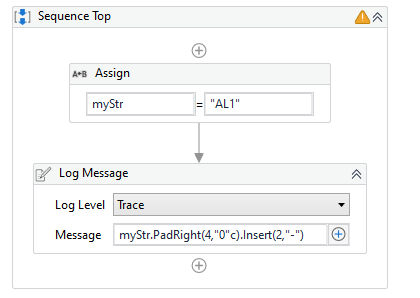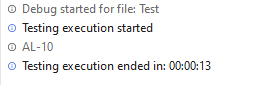# Insert dash between a number and character

So I have a 2 part problem. I need to add a - between characters and numbers as well as make sure the numbers are 2 digits.

EX:

AL1 → AL-01

AL12 → AL-12

These are in a column in an excel.

Not sure where to begin with this. Any help is truly appreciated.

• Assign → Variable1 = “AL12”

Variable1.Insert(2, “-”)

Hope it helps!!

This makes sense. What do i do if it is AL1 and I need to add both the - and a 0?

You can use the below workflow

``````- Assign -> VarText (String) = "AL1"
- Assign -> IndexVarText (int) = VarText.Lenght-1
- If -> IndexVarText>3
- Then -> - Assign -> VarText = VarText.Insert(2,"-")
- Else  -> - Assign -> VarText = VarText.Insert(2,"-0")
``````

Hope you get the solution for your query!!

That will add 0s on the end to make it 4 characters, then insert the - as the third character.thanks all. I actually figured it was easier to do it as an excel formula and have UiPath write it in the dynamic range. i used:

=IF(LEN(P2) = 3, LEFT(P2,2) & “-0” & RIGHT(P2,LEN(P2)-2),LEFT(P2,2) & “-” & RIGHT(P2,LEN(P2)-2))

It’s much better to just write the correct value into Excel than use a formula. That formula is a bit messy and if you need to do other things with this file it could cause issues and difficulty for other users to understand what they’re looking at. Just my \$.02

This topic was automatically closed 3 days after the last reply. New replies are no longer allowed.